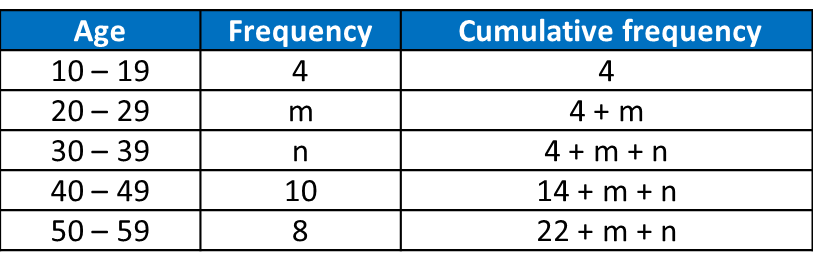# 7.5.1 Statistics Long Questions (Question 1 & 2)

Question 1:
Table shows the age of 40 tourists who visited a tourist spot.Given that the median age is 35.5, find the value of
m and of n.

Solution:
Given that the median age is 35.5, find the value of m and of n.22 + m + n = 40
n = 18 – m —–(1)
Given median age = 35.5, therefore median class = 30 – 39

$\begin{array}{l}35.5=29.5+\left(\frac{20-\left(4+m\right)}{n}\right)×10\\ 6=\left(\frac{16-m}{n}\right)×10\end{array}$
6n = 160 – 10m
3n = 80 – 5m —–(2)

Substitute (1) into (2).
3 (18 – m) = 80 – 5m
54 – 3m = 80 – 5m
2m = 26
m = 13

Substitute m = 13 into (1).
n = 18 – 13
n = 5

Thus m = 13, n = 5.

Question 2:
A set of examination marks x1, x2, x3, x4, x5, x6 has a mean of 6 and a standard deviation of 2.4.
(a) Find
(i) the sum of the marks, $\Sigma x$ ,
(ii) the sum of the squares of the marks, $\Sigma {x}^{2}$ .

(b)
Each mark is multiplied by 2 and then 3 is added to it.
Find, for the new set of marks,
(i) the mean,
(ii) the variance.

Solution:
(a)(i)

(a)(ii)

(b)(i)
Mean of the new set of numbers
= 6(2) + 3
= 15

(b)(ii)
Variance of the original set of numbers
= 2.42 = 5.76

Variance of the new set of numbers
= 22 (5.76)
= 23.04